# OpenTx - Key Concepts

Mike Shellim 25 July 2014
Last updated 15 Oct 2019 - updated section on channels

Para nuestros amigos hispano-hablantes: traducción al español en formato PDF. Muchas gracias a Jorge Brun.

# Introduction

OpenTx is a uniquely flexible system. However if you've come from a brand like Futaba or Spektrum then you'll find the way of working a little unfamiliar. My aim with this article is to shed some light on how it works, and to enable you to design your own setups with confidence.

I'll cover the following topics:

• An overview of OpenTx
• Sources, mixers, inputs and outputs
• A simple methodology for designing setups

Examples will be illustrated either with screenshots or as text boxes, as appropriate.

# Background

OpenTx has just seven programming menus, and each is completely generic. This concept of generic simplicity extends to the mixers. Just like Lego, simple elements are used to build powerful solutions.

But before you can use this power, you'll need to know some of the basics. So let's start at the beginning...

# OpenTx: the processing loop

The core of OpenTx (as with any RC system) is the processing loop. This is a sequence of operations repeated several times a second - fast enough to provide a smooth response.

With each cycle, the position of all the controls are read, mixing is applied, and the commands for each channel are calculated. At the end of the cycle, the channel commands are passed to the RF module for transmission.The three key processing steps are marked in blue. Each has an associated menu ('INPUTS', 'MIXERS' and 'OUTPUTS').

• The INPUTS and MIXERS menus are where you design the control logic (how the system should respond to commands).
• The OUTPUTS menu is where you deal with linkage geometry (setting the centres and limits of each servo channel)

A change at one level propagates to levels lower down.

# The starting point: SourcesLike all computer programs, OpenTx needs some data to work with. In this case the data comes from your transmitter's sticks, trims, knobs and switches. A generic term for these is sources. Each source has a unique identifier, for example,

• Ele = elevator stick
• Thr = throttle stick.
• SA = switch 'SA'
• RS = right slider
• S1 = first knob

The naming of sources is broadly similar between different FrSky transmitters, but there are some differences, e.g. knobs are generally S1 and S2, except on the X9E where they are F1 and F2.

At the start of the processing cycle, OpenTx reads each source, and assigns a value between -100 and 100 according to the position. Zero corresponds to centre; for sticks, left/down is negative; right/up positive. For switches, up is negative, down is positive.

So for example,

• Left slider at centre -> LS = 0
• Elevator stick forward -> Ele = 100
• Aileron stick 50% left -> Ail = -50
• Switch SA down -> SA = 100

Note: channels, Lua scripts and telemetry can also be used as sources, however they will not be considered further in this article.

# Channels and outputsOn most radios, a channel corresponds to a servo output. However in OpenTx, 'channel' has a looser meaning. Key points:

• OpenTx supports up to 32 freely assignable channels.
• The low channels are assigned by the user to servos, ESCs, flight controllers etc, just like any other radio.
• Higher channels may be cascaded to lower channels (advanced programming technique for more complex models).

The OUTPUTS menu is where you define the characteristics of each channel.

For any channel that you want to use, this is where you give the channel a short name.

For the channels driving servos and ESC's, this is where you set the direction, centres and limits. This is normally the last step!

Below is an example screenshot showing a simple RES sailplane. The first three channels have been named 'Rudder', 'Elev' and 'Spoilr'. The travels and centers are still at their default values.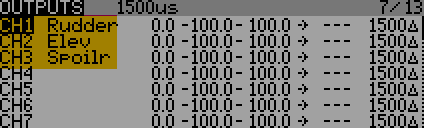Note that the OUTPUTS menu doesn't say how each channel is driven. For that, we need to define some mixers...

# MixersSo far we've talked about channels in isolation. In order to do anything useful, we define some mixers. These act as the 'wiring' from sources and channels; in other words, they define the control logic.

A mixer in OpenTx is a very simple construct:

• ONE mixer represents ONE interaction between ONE source and ONE channel.

A typical setup will involve anything from a couple of mixers (for a simple RE sailplane), to literally dozens of mixers (for a full house F3X ship).

The MIXERS menu is where you can see all your mixers displayed, in channel order. Each non-blank line represents a mixer for that channel. In the screenshot below, the aileron stick drives Channel 1, with 100% effect.Column 1 is the channel number.
Column 2 is the mixer weight expressed as a percentage. 100% means maximum effect, 0% means zero effect. A negative weight reverses the effect.
Column 3 is the mixer source (stick, knob etc)

## Three mixing scenarios

We'll now examine the three basic mixing scenarios. These will form the building blocks of your setup.

### Scenario 1: One source -> one channel

This is the simplest scenario. We've already looked at an example where the aileron stick drives CH1. 100% of the aileron command is passed to the output:### Scenario 2: One source -> multiple channels

In this scenario, a single source drives more than one channel. In the example below, the aileron stick drives both CH1 and CH5. This is a popular configuration for dual ailerons: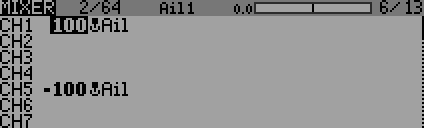This configuration emulates a Y-lead but is more flexible since each channel can be individually adjusted for direction, travel and centring via the OUTPUTS menu.

### Scenario 3: Multiple sources -> one channel

A single channel can also be driven by multiple sources simultaneously. A common example of this is a V-tail channel - the surface is driven by the rudder and elevator sticks: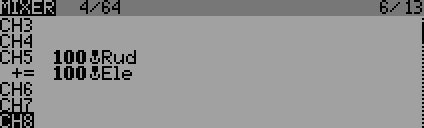Note the '+' sign against the Ele line, this indicates that the rudder and elevator inputs are added to produce the output. When using the add operator, the order of the mixers doesn't matter. Other operators like multiply and replace can also be used, and the order for these is important (we'll look at those later).

# Designing a mixer scheme

Now you understand how mixers work, let's build a complete working system. We'll use a a simple 6-step method which can be applied regardless of complexity.

We'll use the example of a 2-channel flying wing. While very simple, it shows the elegance and power of OpenTx's mixing model.

### Step 1. List the sources

The first step is to list the sources, in other words the sticks and sliders used to control the model. Don't include flight mode switches or rate switches.

Our flying wing uses aileron and elevator sticks, so the list of sources is:

• Ail
• Ele

### Step 2. List the servo channels

Now assign the servo channels.

Our flying wing has two elevon servos, so we assign two channels named 'RtEvon' and 'LtEvon'. We do this directly in the OUTPUTS menu: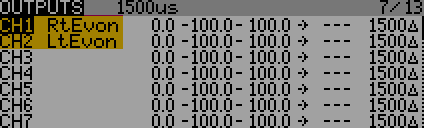### Step 3. Identify interactions

Now identify all the interactions between sources and channels, and list them in the form source->channel. We're not interested in how the channels are affected, just the fact that the interactions exist.

For our flying wing, the Ail stick drives channels CH1 and CH2. So:

• Ail -> CH1
• Ail -> CH2

Similarly, the interactions for Ele are:

• Ele -> CH1
• Ele -> CH2

### Step 4: Convert interactions into mixer definitions

Next, swap the left and right sides of each interaction so that they read as "channel is affected by source".

• CH1 <- Ail
• CH2 <- Ail
• CH1 <- Ele
• CH2 <- Ele

### Step 5: Reorder interactions by channel number

Now, re-order the mixer definitions so that they're grouped by channel.

• CH1 <- Ail
• CH1 <- Ele
• CH2 <- Ail
• CH2 <- Ele

### Step 6: Enter definitions into the MIXER menu

Finally, enter the interactions as mixer definitions:The '+=' means on each line shows that the mixes are additive.

Once you've done this a few times, you'll be able to do it in your head. But I still recommend using Companion for your first experiments, and for complex setups - mistakes are much easier to debug on a big screen.

# Mixer weights

The mixer weight determines the effect, or rate, of the mix. Weight is specified as a percentage - zero means no effect, 100% means maximum effect, a negative value reverses the effect.

Let's illustrate this with our flying wing. Typically, roll commands require more elevon movement than pitch. We therefore set a higher weight for the Ail mixes:Note that the Ail weight is negative for one of the mixes and positive in the other. This is because the elevons move in opposite directions in response to aileron commands.

Our flying wing setup works, but it has a shortcoming: in order to adjust rates, we have to alter the mixer weight in two places - that's double the work, and double the chance of error. Always avoid duplication!We can avoid duplicates by using Inputs (introduced in OpenTx v. 2.0).

## INPUTSAn input is essentially just a regular source, but with weight and expo included. As you can see from the heading diagram Inputs are optional, however it's good practice to use inputs for the main flight controls.

Inputs are distinguished from regular sources by a leading '[I]'. Using our flying wing example, we'll define a couple of inputs for the Ail and Ele controls.

2. Name the first input as '[I]Ail' and set the source to Ail.
3. Name the second input as '[I]Ele' and set the source to Ele.
4. Finally, set weight and expo to the required values

This is how it looks in the menu: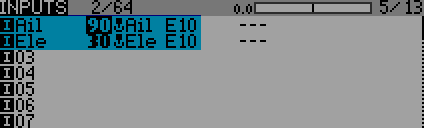[I]Ail and [I]Ele behave exactly like the raw sources ail and ele, except the inputs have reduced weight and 10% expo.

Returning to the flying wing example, we amend the mixer definitions to use inputs.

Note that the mixer weights are reset to +/-100%!! This is good practice, as it localises the rate adjustment to just the INPUTS menu.The OUTPUTS menu remains unchanged - for now (we'll come back to it later when calibrating the servos):### Choosing between raw sources and inputs

Inputs are optional, and it doesn't always make sense to use them.

• Use inputs for the main flight controls e.g. rudder, elevator, aileron sticks. This will allow you to alter rate and expo easily.
• Use the raw sources for controls such as motor, spoiler, crow. These typically don't require adjustable rates or expo - adjustment to these willl normally be made in the Outputs menu.

Inputs are an important part of OpenTx, and you can read more about them here.

# How mixer and channel outputs are calculatedIn this section we'll look in detail at how OpenTx converts stick movements into position commands. An understanding will be come in useful when debugging your setup.

### Mixer output values

OpenTx performs two stages of calculation, first at the level of the mixers, and then for the channel as a whole.

For each mix, OpenTx calculates the mixer output according the weight (and other parameters we'll look at later). Taking our flying wing example, at any moment the mixer outputs are as follows:

Channel 1

mix_1 = Ail_stick_value x 90%

mix_2 = Ele_stick_value x 30%

Channel 2

mix_1 = Ail_stick_value x -90%

mix_2 = Ele_stick_value x 30%

### Channel values

OpenTx then aggregates the mixer outputs for each channel. The result is the channel output value. This represents the commanded position. Using our flying wing:

ch1 = (Ail_stick_val x 90%)+(Ele_stick_val x 30%)
ch2 = (Ail_stick_val x -90%)+(Ele_stick_val x 30%)

Here are the numbers for our flying wing example:

 S T I C K S C H A N N E L S Ail stick Ele stick CH1 CH2 (centre) 0 (centre) 0 0 0 (full  right) 100% (centre) 0 90+0=90 -90+0=-90 (full left) -100% (centre) 0 -90+0=-90 90+0=90 (centre) 0 (full forward) 100% 0+30=30 0+30=30 (half right) 50% (half forward) 50% 45+15=60 -45+15=-30 (full  right) 100% (full forward) 100% 90+30=120 -90+30=-60

The last line shows the effect of "stick in the corner" - note the commanded position for Channel 1 is 120. In fact, OpenTx clips the channel values to +/- 100 in the OUTPUTS section - this ensures that servo travel is confined within safe bounds. More on that later.OpenTx offers a number of mechanisms for conditioning the output of a mix. We've already looked at weight. We'll now investigate offset, expo, diff, functions and curves.

### Mixer offset

The offset parameter is used to shift the mixer output up or down. Using offset in conjunction with weight, you can create mixes whose output varies between a defined range. This is useful for creating volume controls, and also for compensation mixes.

When calculating the output of a mixer, offset is applied after weight:

Mixer output = (Source * weight) + Offset

Offset example 1: custom volume control

Suppose you want to create volume control (S1), with output varying from -50 to +90 as S1 is rotated. We can do this as follows (remembering that the value of S1 varies from -100 to +100):

Src = 'S1', wt=70%, offset=20

Offset example 2: motor-elevator compensation. Offsets are commonly used for crow and motor mixes, in particular 'compensation' mixes. For these mixes, the output must be zero at the idle end of stick travel.

The example below shows a typical motor-elevator mix. The first line is the standard elevator mix, the second line is for motor compensation.

CH2 (elevator)

Src=Ele, wt=100%, offset = 0

Src=Thr, wt=20%, offset=20

Note how weight and offset are the same value - this ensures that the output is zero when the throttle stick is at back/idle (-100). At full throttle (+100) the output will be 2 * weight = 40. To reverse the stick, change the sign of weight.

Note: offsets should be used sparingly! Never use them to make ad-hoc adjustments to your servo centres or end points - use the OUTPUTS menu for that.

### Diff, expo, functions and curves

In addition to weight and offset, OpenTx provides one additional option from the following:

• Diff
• Expo
• Function (e.g. x > 0)
• Curve

The 'Curve' option provides the most granularity - it can implement all the other options, but requires the most data entry.

### Using the 'diff' option

Sailplanes often employ aileron differential. The purpose is to reduce the travel of the downgoing aileron. First, here's the obvious - but incorrect - way:

[I1]Ail

Src=Ail, wt=90%, expo=10, diff=20

CH1 (right elevon)

Src = [I1], wt=100%

CH2 (left elevon)

Src = [I1], wt=-100%

The problem: diff is applied to the input, so the aileron stick will have a higher rate on one side than the other. This is not what we want! Instead, diff must be applied separately to each aileron channel:

[I1]Ail

Src=Ail, wt=90%, expo=10, diff=0

CH1 (right elevon)

Src = [I1], wt=100%, diff=20%

CH2 (left elevon)

Src = [I1], wt=-100%, diff=20%

To summarise: specify diff separately for each aileron channel. Do not specify diff at the Input level.

TIP: Use a GVAR to supply the diff value. That way, you can set diff for both channels with a single adjustments.

### Including/excluding trims

By default, trim values are included in sources. You can exclude trims on a per-mixer basis by unchecking "Include Trim" in the mixer dialog.

For our flying wing example, aileron and elevator trims must always be active, so we'll use the default settings.

### Default mixer settings

When you create a new mix, the initial settings are weight=100% and offset=0, diff/expo=0 and no curve. The source value is therefore passed through unchanged.

### Order of processing

When calculating the output of a mix, OpenTx first applies the trim value. Then it applies the function (diff, function, curve, expo), followed by weight, and finally adds the offset. Order may be important when combining operators especially where offsets are involved.In the final step of the processing loop, OpenTx clips and scales the outputs. You manage this in the OUTPUTS menu. This is where you'll match the outputs to the servo and linkage characteristics.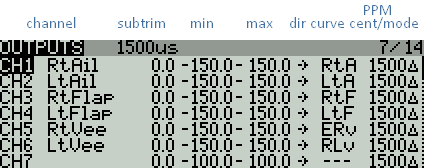Clipping

If several mixers are active, then the channel value could exceed the safe limits of the servo.

CHxOutput = SUM (CHxMixerOutput1, CHxMixerOutput2, ...)

To avoid this, channel values are clipped in the OUTPUTS stage so they lie in the range -100 to +100. Clipping manifests itself as deadband at the extremes of stick movement.

Scaling and offset

Next, the channel commands are scaled and offset. This is how you map channel values to actual servo actual positions.

First, an optional curve is applied (specified in the Curve field). Curves may be between 2 and 17 points, and provide fine grained control over the channel response.

Next, the command is scaled according to MIN, MAX and SUBTRIM. Think of these as a three point curve defining the end points and centre.

Notes:

• MIN/MAX/SUBTRIM are applied even if a curve has been specified. To avoid confusion when using a curve, it's good practice to leave MIN/MAX/SUBTRIM at 'pass-thru' values (-100/100/0, or -150/150/0 if using extended limits). That way, the curve can be adjusted without consideration to the MIN/MAX/SUBTRIM settings.
• By default, the maximum values for MIN and MAX are -100% and +100%. You can expand this to -150/+150% by specifying Extended Limits in the MODEL SETUP menu.
• A good way of calibrating the servo limits is to generate -100 and +100 channel values, then adjust MIN and MAX for the required servo limits. (Or, if using a curve, adjust the curve end points.)
• Similarly, you calibrate servo centres by generating a channel value of zero, then adjusting SUBTRIM to suit (or, if using a curve, adjust the mid point).

The three processing layers (Inputs, Mixers, and Outputs) apply a weight to their inputs. As a source value propagates through these layers, each rate is applied to the result of the previous layer. The the final servo command will be the product of at least three rates:

OutputValue = SourceValue x Rateinput x Ratemix x Rateoutput

Clearly there is an infinite combination of rates which will produce the same movement at the servo. So where should you set them? This is the procedure I recommend:

1. Initialise all weights in MIXERS and INPUTS to 100%.
2. Calibrate the servo centres and end points. To do this, go to the OUTPUTS menu. Move each control to one or other end stop and adjust MIN/MAX to set the limits of travel. Then adjust SUBTRIM for correct centres.
3. The control surface movements after step (2) will be excessive. To achieve the movements required for flight, adjust the weights in the INPUTS menu.
4. Any remaining mixer interactions can be adjusted via weights in the MIXERS menu.

## Mixer operators

So far, we've assumed that mixer outputs are always added together. In fact OpenTx is a good deal more flexible - it also permits multiplication and replace operations. This is very useful. For example, multiplication is key to implementing in-flight adjusters, and 'replace' can be used to implement throttle safety switches.

The key is the mixer's multiplex parameter. This defines how the mixer's value should update the channel value. The options are 'ADD' (the default), 'REPL' and 'MULT'.

When calculating a channel value, OpenTx initially assigns a value of zero. It then steps through all the mixers in the channel, starting from the top. Processing of each mix depends on the multiplex parameter:

• ADD - the mixer value is added to the channel value.
• REPL - the mixer value replaces the channel value.
• MULT - the channel value is multiplied by the mixer value, and the result becomes the new channel value

Note:

• Channel outputs are zero by default
• For REPL or MULT ops, the order of the mixer lines is important.
• If a mix is disabled (e.g. via a switch), its ignored.

The following examples illustrate the effect of the various multiplex options. Note the importance of mixer order when using 'REPL' and 'MULT'.

output = I1 + I2

Src = I3, op=MULT

output = (I1 + I2) * I3

Src = I2, op=MULT

output = (I1 * I2) + I3

Src = I2, op=MULT (disabled)

output = I1 + I3

Src = I2, op=REPL

output = I2 + I3

Src = I2, op=REPL

Src = I3, op=MULT

output = I2 * I3

output = 0

## The 'MAX' source

MAX is a special source which doesn't correspond to a physical control. Instead, MAX supplies a fixed value of +100. In conjunction with weight, it can be used to simulate the effect of a fixed stick position.

Src = MAX, wt =100 -- output = 100%

Src = MAX, wt=50 -- output = 50%

Src = MAX, wt=-100 -- output = -100%

Here's a simple example, showing a crude motor arming system. The motor is armed when SA is down.

• The Thr line provides variable motor control via the throttle stick
• The MAX line provides a fixed value of -100 corresponding to motor off. The line becomes active when SA is not in the down position (the ! operator in !SA_down means 'not'). When it's active, it overrides the previous line (via the 'multiplex=REPLACE' parameter), and stops the motor.

CH7 (motor)

Src = Thr, wt=100%,

Src = MAX, wt=-100%, switch=!SA_down, multiplex = REPL

## F3X sailplane mixer scheme

If you're familiar with F3X sailplanes, you may wish to look at interactions and mixes for F3X sailplanes. Such a scheme will normally be optimised by means of Inputs (described later in this article), GVARs and cascading mixers.